# NCERT Solutions for Class 10 Maths Exercise 1.1## myCBSEguide App

Complete Guide for CBSE Students

NCERT Solutions, NCERT Exemplars, Revison Notes, Free Videos, CBSE Papers, MCQ Tests & more.

NCERT solutions for Class 10 Maths Real Numbers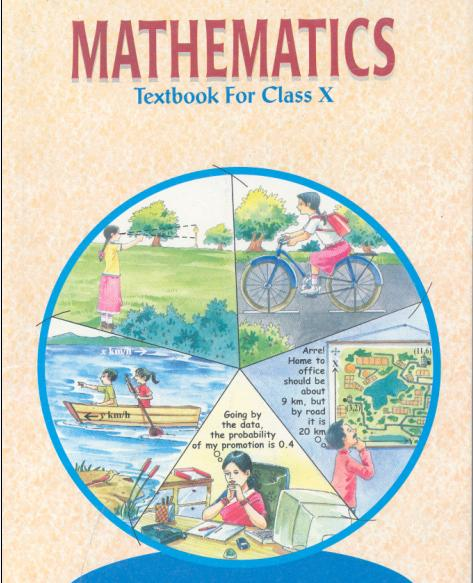## NCERT Solutions for Class 10 Maths Real Numbers

###### 1. Use Euclid’s division algorithm to find the HCF of:

(i) 135 and 225

(ii) 196 and 38220

(iii) 867 and 255

Ans. (i) 135 and 225

We have 225 > 135,

So, we apply the division lemma to 225 and 135 to obtain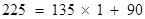Here remainder 90 ≠ 0, we apply the division lemma again to 135 and 90 to obtain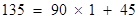We consider the new divisor 90 and new remainder 45 ≠ 0, and apply the division lemma to obtain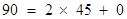Since that time the remainder is zero, the process get stops.

The divisor at this stage is 45

Therefore, the HCF of 135 and 225 is 45.

(ii) 196 and 38220

We have 38220 > 196,

So, we apply the division lemma to 38220 and 196 to obtain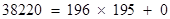Since we get the remainder is zero, the process stops.

The divisor at this stage is 196,

Therefore, HCF of 196 and 38220 is 196.

(iii) 867 and 255

We have 867 > 255,

So, we apply the division lemma to 867 and 255 to obtain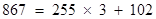Here remainder 102 ≠ 0, we apply the division lemma again to 255 and 102 to obtain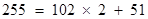Here remainder 51 ≠ 0, we apply the division lemma again to 102 and 51 to obtain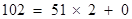Since we get the remainder is zero, the process stops.

The divisor at this stage is 51,

Therefore, HCF of 867 and 255 is 51.

NCERT Solutions for Class 10 Maths Exercise 1.1

###### 2. Show that any positive odd integer is of the form 6q + 1, or 6q + 3, or 6q + 5, where q is some integer.

Ans. Let a be any positive integer and b = 6. Then, by Euclid’s algorithm,

a = 6q + r for some integer q0, and r = 0, 1, 2, 3, 4, 5 because 0 r < 6.

Therefore, a = 6q or 6q + 1 or 6q + 2 or 6q + 3 or 6q + 4 or 6q + 5

Also, 6q + 1 =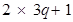=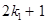, where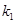is a positive integer

6q + 3 = (6q + 2) + 1 = 2 (3q + 1) + 1 =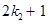, where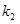is an integer

6q + 5 = (6q + 4) + 1 = 2 (3q + 2) + 1 =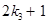, where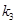is an integer

Clearly, 6q + 1, 6q + 3, 6q + 5 are of the form 2k + 1, where k is an integer.

Therefore, 6q + 1, 6q + 3, 6q + 5 are not exactly divisible by 2. Hence, these expressions of numbers are odd numbers.

And therefore, any odd integer can be expressed in the form 6q + 1, or 6q + 3,

or 6q + 5

NCERT Solutions for Class 10 Maths Exercise 1.1

###### 3. An army contingent of 616 members is to march behind an army band of 32 members in a parade. The two groups are to march in the same number of columns. What is the maximum number of columns in which they can march?

Ans. We have to find the HCF (616, 32) to find the maximum number of columns in which they can march.

To find the HCF, we can use Euclid’s algorithm.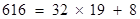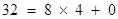The HCF (616, 32) is 8.

Therefore, they can march in 8 columns each.

NCERT Solutions for Class 10 Maths Exercise 1.1

###### 4. Use Euclid’s division lemma to show that the square of any positive integer is either of form 3m or 3m + 1 for some integer m. [Hint: Let x be any positive integer then it is of the form 3q, 3q + 1 or 3q + 2. Now square each of these and show that they can be rewritten in the form 3m or 3m + 1.]

Ans. Let a be any positive integer and b = 3.

Then a = 3q + r for some integer q ≥ 0

And r = 0, 1, 2 because 0 ≤ r < 3

Therefore, a = 3q or 3q + 1 or 3q + 2

Or,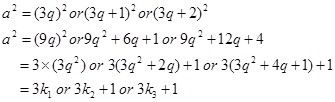Where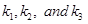are some positive integers.

Hence, it can be said that the square of any positive integer is either of the form 3m or 3m + 1.

NCERT Solutions for Class 10 Maths Exercise 1.1

###### 5. Use Euclid’s division lemma to show that the cube of any positive integer is of the form 9m, 9m + 1 or 9m + 8.

Ans. Let a be any positive integer and b = 3

a = 3q + r, where q ≥ 0 and 0 ≤ r < 3

\ a = 3q or 3q + 1 or 3q + 2

Therefore, every number can be represented as these three forms.

We have three cases.

Case 1: When a = 3q,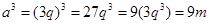Where m is an integer such that m =3q3

Case 2: When a = 3q + 1,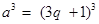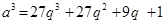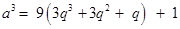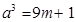Where m is an integer such that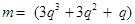Case 3: When a = 3q + 2,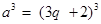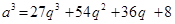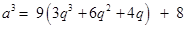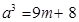Where m is an integer such that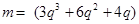Therefore, the cube of any positive integer is of the form 9m, 9m + 1, or 9m + 8.

## NCERT Solutions for Class 10 Maths Exercise 1.1

NCERT Solutions Class 10 Maths PDF (Download) Free from myCBSEguide app and myCBSEguide website. Ncert solution class 10 Maths includes text book solutions from Mathematics Book. NCERT Solutions for CBSE Class 10 Maths have total 15 chapters. 10 Maths NCERT Solutions in PDF for free Download on our website. Ncert Maths class 10 solutions PDF and Maths ncert class 10 PDF solutions with latest modifications and as per the latest CBSE syllabus are only available in myCBSEguide.

## CBSE app for Class 10

To download NCERT Solutions for Class 10 Maths, Computer Science, Home Science,Hindi ,English, Social Science do check myCBSEguide app or website. myCBSEguide provides sample papers with solution, test papers for chapter-wise practice, NCERT solutions, NCERT Exemplar solutions, quick revision notes for ready reference, CBSE guess papers and CBSE important question papers. Sample Paper all are made available through the best app for CBSE students and myCBSEguide website.## Test Generator

Create Papers with your Name & Logo

### 8 thoughts on “NCERT Solutions for Class 10 Maths Exercise 1.1”

1. Nice solution

2. Helps students to do thir home work alot

3. good guide

4. this is brilliant

5. This is awesome and billiant

6. jabarjast solution

7. Nice sir

8. Perfect solution